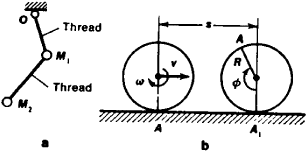# Holonomic Systems

The following article is from The Great Soviet Encyclopedia (1979). It might be outdated or ideologically biased.

## Holonomic Systems

mechanical systems in which all links are geometrical (holonomic)—that is, restricting the position (or displacement during motion) of points and bodies in the system but not affecting the velocities of these points and bodies. For example, the double pendulum in Figure 1, a is a holonomic system, in which the links (threads) restrict the position or displacement of the weights M, and M2 but not their velocity, which can have any value.Figure 1

Links that restrict the velocities of points and bodies in a system (that is, links that establish specific ratios between these velocities) are called kinematic. However, if the ratios in such a system are geometrical—that is, if the relationships between displacements of points and bodies in the system are geometrical—the system is also holonomic. For example, when a wheel with radius R rolls along a straight rail without sliding (Figure l.b), the velocity v of the center of the wheel and the angular velocity ω of the wheel are related by the equation v = R ω, but it is possible to reduce it to the geometrical correspondence S = Rф between the displacement of the center (s = AA1,) and the angle of rotation ø of the wheel. Thus, this system is holonomic.

Kinematic links that cannot be reduced to geometrical links are called nonholonomic, and mechanical systems that use such links are called nonholonomic systems. The division of mechanical systems into holonomic and nonholonomic is most significant, since a number of equations that make possible the comparatively simple solution of mechanical problems are applicable only to holonomic systems.

S. M. TARG